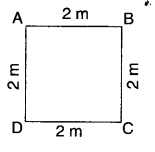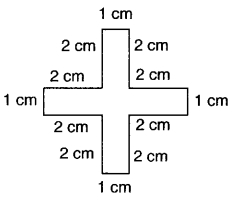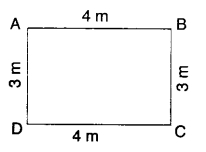# CBSEtips.in

## Sunday 28 February 2021

### CBSE Class 6 Maths - MCQ and Online Tests - Unit 10 - Mensuration

#### CBSE Class 6 Maths – MCQ and Online Tests – Unit 10 – Mensuration

Every year CBSE students attend Annual Assessment exams for 6,7,8,9,11th standards. These exams are very competitive to all the students. So our website provides online tests for all the 6,7,8,9,11th standards’ subjects. These tests are also very effective and useful for those who preparing for any competitive exams like Olympiad etc. It can boost their preparation level and confidence level by attempting these chapter wise online tests.

These online tests are based on latest CBSE syllabus. While attempting these, our students can identify their weak lessons and continuously practice those lessons for attaining high marks. It also helps to revise the NCERT textbooks thoroughly.#### CBSE Class 6 Maths – MCQ and Online Tests – Unit 10 – Mensuration

Question 1.
Apala went to a park 20 m long and 10 m wide. She took one complete round of it. The distance covered by her is
(a) 30 m
(b) 60 m
(c) 20 m
(d) 10 m.

Hint:
Distance covered = 2(20 + 10) = 60 m

Question 2.
The perimeter of the figure is(a) 8m
(b) 16m
(c) 4m
(d) none of these.

Hint:
Perimeter = 4 × 2 = 8m

Question 3.
Perimeter of a square =
(a) 4 × Length of a side
(b) 2 × Length of a side
(c) 3 × Length of a side
(d) 6 × Length of a side.

Question 4.
A page is 25 cm long and 20 cm wide. Find the perimeter of this page.
(a) 90 cm
(b) 45 cm
(c) 500 cm
(d) 5 cm.

Hint:
Perimeter = 2(25 + 20) = 90 cm

Question 5.
Perimeter of a regular hexagon =
(a) 3 × Length of a side
(b) 4 × Length of a side
(c) 5 × Length of a side
(d) 6 × Length of a side.

Question 6.
The perimeter of the figure is(a) 5 cm
(b) 10 cm
(c) 15 cm
(d) 20 cm.

Hint:
Perimeter = 4(1 + 2 + 2) = 20cm

Question 7.
Perimeter of a rectangle =
(c) 2 × (Length + Breadth)
(d) 2 × (Length × Breadth).

Question 8.
The perimeterof the figure is(a) 20 cm
(b) 10 cm
(c) 24 cm
(d) 15 cm.

Hint:
Perimeter = 4 + 3 + 2 + 2 + 3 + 6 = 20 cm

Question 9.
Meenu wants to put a lace border all around a rectangle table cover 2 m long and 1 m wide. Find the length of the lace required by Meenu.
(a) 3 m
(b) 4 m
(c) 5 m
(d) 6m.

Hint:
Length of the lace = 2(2 + 1) = 6 m

Question 10.
Perimeter of an equilateral triangle
(a) 2 × Length of a side
(b) 3 × Length of a side
(c) 4 × Length of a side
(d) 6 x Length of a side.

Question 11.
Find the perimeter of a rectangle whose length and breadth are 9 cm and 1 cm respectively,
(a) 10 cm
(b) 20 cm
(c) 30 cm
(d) 40 cm.

Hint:
Perimeter = 2(9 + 1) = 20 cm

Question 12.
An athlete takes 10 rounds of a rectangular park, 40 m long and 30 m wide. Find the total distance covered by him.
(a) 1400 m
(b) 700 m
(c) 70 m
(d) 2800 m.

Hint:
Total distance covered = 10 × 2 × (40 + 30) = 1400 m

Question 13.
The perimeter of a regular pentagon is 10 m. Find the length of the side.
(a) 1m
(b) 2m
(c) 5m
(d) 10m

Hint:
Length of side = $$\frac { 10 }{ 5 }$$ = 2 m

Question 14.
Find the cost of fencing a rectangular park of length 10 m and breadth 5 m at the rate of? 10 per metre.
(a) ₹ 300
(b) ₹ 600
(c) ₹ 150
(d) ₹ 1200.

Hint:
Cost= 10 × 2 × (10 + 5) = ₹ 300

Question 15.
Area of a rectangle =
(c) 2 × (Length + Breadth)
(d) 2 × (Length × Breadth).

Question 16.
The perimeter of a square of side 1 m is
(a) 1 cm
(b) 2 cm
(c) 3 cm
(d) 4 m.

Hint:
Perimeter = 4 × 1 =4m

Question 17.
Area of a square =
(a) side × side
(b) 4 × Length of a side
(c) 2 × Length of a side
(d) 6 × Length of a side.

Question 18.
The perimeter of an equilateral triangle of side 1 m is
(a) 1 m
(b) 2 m
(c) 3 m
(d) 6 m.

Hint:
Perimeter = 3 × 1 = 3 m

Question 19.
The perimeter of a regular pentagon of side 1 m is
(a) 5 m
(b) 10 m
(c) 15m
(d) 20m.

Hint:
Perimeter = 5 × 1 = 5 m

Question 20.
The perimeter of an equilateral triangle is 9 m. Find the length of the side.
(a) 1 m
(b) 2 m
(c) 3 m
(d) 9 m.

Hint:
Length of side = $$\frac { 9 }{ 3 }$$ = 3 m

Question 21.
Perimeter of a regular pentagon =
(a) 4 × Length of a side
(b) 3 × Length of a side
(c) 6 × Length of a side
(d) 5 × Length of a side.

Question 22.
The perimeter of a regular hexagon of side 1 m is
(a) 3 m
(b) 2 m
(c) 4 m
(d) 6 m.

Hint:
Perimeter = 6 × 1 = 6m

Question 23.
The perimeter of the figure is(a) 12m
(b) 14m
(c) 24 m
(d) 7 m.

Hint:
Perimeter = 2(4 + 3) = 14m

Question 24.
Find the distance travelled by Sangeeta if she takes 5 rounds of a square park of side 10 m.
(a) 200 m
(b) 100 m
(c) 400m
(d) 800 m.

Hint:
Distance = 5 × 4 × 10 = 200 m

Question 25.
The perimeter of a square is 8 m. Find the length of the side.
(a) 1m
(b) 2m
(c) 4m
(d) 8m.

Hint:
Length of side = $$\frac { 8 }{ 4 }$$ = 2 m

Share: